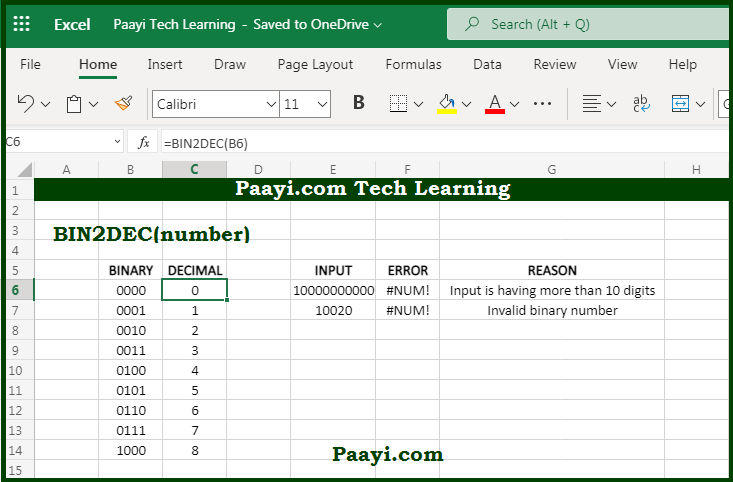# Learn How to Use Microsoft Excel BIN2DEC Function

Written by | 0 Comments | 618 Views

In this article, you will learn how to use the Microsoft Excel BIN2DEC function and its prime function in Microsoft Excel. You will also get to know the Microsoft Excel BIN2DEC function return value and syntax with the help of some examples.

Microsoft Excel BIN2DEC Function

The main function of the Microsoft Excel BIN2DEC function is used to convert binary numbers into decimals. So, with the help of the BIN2DEC function, you can able to convert a binary number to its decimal equivalent. The input numbers should contain zeroes and ones and be less than ten characters long; otherwise, the BIN2DEC function will return the #NUM! Error value.

Return Value of BIN2DEC Function

The return value will be the decimal equivalent of the binary number.

Syntax of BIN2DEC Function

=BIN2DEC(number)

Where the argument:

number: It is the binary numbers you want to convert to decimals.

## How to Use Microsoft Excel BIN2DEC Function?The first bit of binary number represents whether the number is negative or positive, while the remaining nine digits represent the size of the number. The maximum can be 511 (2^9 - 1). The negative binary number is expected to use the two's complement notation.